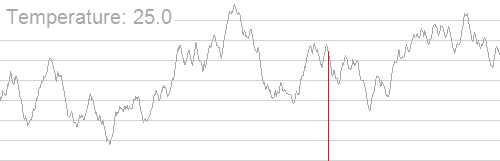# 模拟退火

## 解释

### 过程### 如何退火（降温）？## 实现

  1 2 3 4 5 6 7 8 9 10 11 12 13 14 15 16 17 18 19 20 21 22 23 24 25 26 27 28 29 30 31 32 33 34 35 36 37 38 39 40 41 42 43 44 45 46 47 48 49 50 #include #include #include #include const int N = 10005; int n, x[N], y[N], w[N]; double ansx, ansy, dis; double Rand() { return (double)rand() / RAND_MAX; } double calc(double xx, double yy) { double res = 0; for (int i = 1; i <= n; ++i) { double dx = x[i] - xx, dy = y[i] - yy; res += sqrt(dx * dx + dy * dy) * w[i]; } if (res < dis) dis = res, ansx = xx, ansy = yy; return res; } void simulateAnneal() { double t = 100000; double nowx = ansx, nowy = ansy; while (t > 0.001) { double nxtx = nowx + t * (Rand() * 2 - 1); double nxty = nowy + t * (Rand() * 2 - 1); double delta = calc(nxtx, nxty) - calc(nowx, nowy); if (exp(-delta / t) > Rand()) nowx = nxtx, nowy = nxty; t *= 0.97; } for (int i = 1; i <= 1000; ++i) { double nxtx = ansx + t * (Rand() * 2 - 1); double nxty = ansy + t * (Rand() * 2 - 1); calc(nxtx, nxty); } } int main() { srand(0); // 注意，在实际使用中，不应使用固定的随机种子。 scanf("%d", &n); for (int i = 1; i <= n; ++i) { scanf("%d%d%d", &x[i], &y[i], &w[i]); ansx += x[i], ansy += y[i]; } ansx /= n, ansy /= n, dis = calc(ansx, ansy); simulateAnneal(); printf("%.3lf %.3lf\n", ansx, ansy); return 0; }